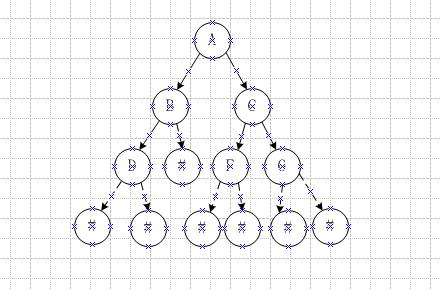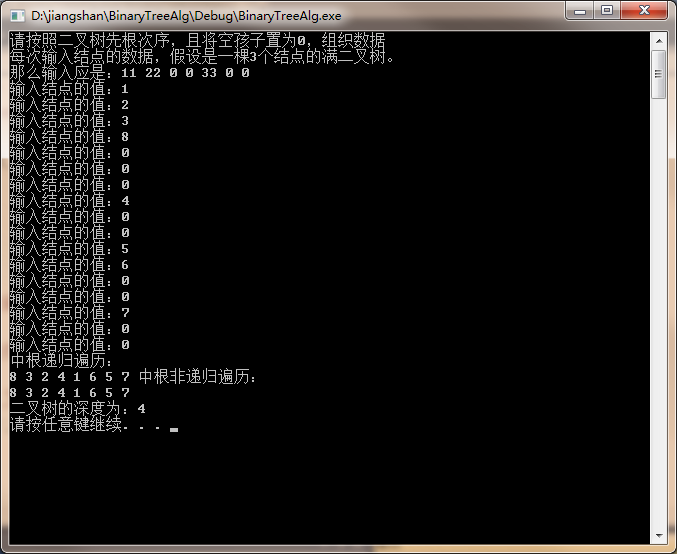• 链表实现二叉树创建和遍历，三种遍历方式实现，二叉树
• ## 二叉树建立和遍历

千次阅读 2014-07-24 15:47:04
二叉树建立和遍历
二叉树创建遍历规则:
1.先序:根-左-右
2.中序:左-根-右
3.后序:左-右-根

二叉树定义和辅助函数如下：

struct node {
int data;
struct node* left;
struct node* right;
};

void visit(int data)
{
printf("%d ", data);
}

int indata()
{
int data;
scanf("%d",&data);
return data;
}

先序创建二叉树:

struct node* CreateBiTree()//先序建立一个二叉树
{
char x; //x为根节点
struct node* t;
x=indata();
if (x==' ') /* 判断当前子树是否创建完成*/
return NULL;
else
{
t=(struct node*)malloc(sizeof(struct node));
t->data=x;
t->left=CreateBiTree();
t->right=CreateBiTree();
}
return t;
}  
先序遍历二叉树：

void preOrder(struct node* root)
{
if (root == NULL)
return;
visit(root->data);
preOrder(root->left);
preOrder(root->right);
}


中序创建二叉树:

struct node* CreateBiTree()//先序建立一个二叉树
{
char x; //x为根节点
struct node* t;
x=indata();
if (x==' ') /* 判断当前子树是否创建完成*/
return NULL;
else
{
t=(struct node*)malloc(sizeof(struct node));
t->left=CreateBiTree();
t->data=x;
t->right=CreateBiTree();
}
return t;
}  
中序遍历二叉树:

void inOrder(struct node* root)
{
if (root == NULL)
return;
inOrder(root->left);
visit(root->data);
inOrder(root->right);
}  

后序创建二叉树

struct node* CreateBiTree()//先序建立一个二叉树
{
char x; //x为根节点
struct node* t;
x=indata();
if (x==' ') /* 判断当前子树是否创建完成*/
return NULL;
else
{
t=(struct node*)malloc(sizeof(struct node));
t->left=CreateBiTree();
t->right=CreateBiTree();
t->data=x;
}
return t;
}

后序遍历二叉树

void inOrder(struct node* root)
{
if (root == NULL)
return;
inOrder(root->left);
inOrder(root->right);
visit(root->data);
}  

转载请注明作者：小刘


展开全文• 二叉树创建遍历，使用递归调用，含前序遍历、中序遍历、后序遍历、层序遍历
• 大型二叉树建立遍历系统\大型二叉树建立遍历系统.
• 描述 题目描述 ...建立起此二叉树以后，再对二叉树进行中序遍历，输出遍历结果。 输入描述: 输入包括1行字符串，长度不超过100。 输出描述: 可能有多组测试数据，对于每组数据， 输出将输入字符...
描述
题目描述  编一个程序，读入用户输入的一串先序遍历字符串，根据此字符串建立一个二叉树（以指针方式存储）。 例如如下的先序遍历字符串： ABC##DE#G##F### 其中“#”表示的是空格，空格字符代表空树。建立起此二叉树以后，再对二叉树进行中序遍历，输出遍历结果。  输入描述:  输入包括1行字符串，长度不超过100。  输出描述:  可能有多组测试数据，对于每组数据，  输出将输入字符串建立二叉树后中序遍历的序列，每个字符后面都有一个空格。  每个输出结果占一行。
示例1

输入  abc##de#g##f###  输出  c b e g d f a

分析
就是根据前序字符串建立二叉树，然后再中序遍历。关键在建立，新建树的BitNode类型，然后递归建树
代码
#include<iostream>
#include<cstdio>
#include<algorithm>
#include<cstring>
#include<string>
char tree;
using namespace std;
typedef struct node {
char data;
struct node * lchild;
struct node * rchild;
}BitNode;
int len = 0;
int pos = 0;
BitNode* createTree()
{
if (pos >= strlen(tree))return NULL;
if (tree[pos] == '#') { pos++; return NULL; }
else {
BitNode * T = new BitNode;
T->data= tree[pos++];
T->lchild = createTree();
T->rchild = createTree();
return T;
}
}
void Inorder(BitNode*T)
{
if (T != NULL)
{
Inorder(T->lchild);
cout << T->data<<" ";
Inorder(T->rchild);
}
}
int main()
{
while (~scanf("%s", tree))
{
pos = 0;
BitNode * T = createTree();
Inorder(T);
cout << "\n";
}
}
补充
前序遍历：
void PreOrder(BitNode * T)
{
if(T!=NULL){
cout<<T->data<<" ";
PreOrder(T->lchild);
PreOrder(T->rchild);
}
}

后序遍历
void PostOrder(BitNode * T)
{
if(T!=NULL){
PostOrder(T->lchild);
PostOrder(T->rchild);
cout<<T->data<<" ";
}
}
以广义表的形式输出二叉树：
void printBinTree(BitNode * T){
if(T!=NULL)
{
cout<<T->data;
if(T->lchild!=NULL || T->rchild!=NULL)
{  cout<<'(';
printBinTree(T->lchild);
cout<<',';
if(T->rchild!=NULL)printBinTree(T->rchild);
cout<<')';
}
}
}
计算一颗二叉树的高度
int Height(BitNode * T)
{
if(T==NULL) return 0;
else{
int i = Height(T->lchild);
int j = Height(T->rchild);
if(i<j)return j+1;
else return i+1
}
}
一些性质：  1、二叉树的第i层，最多有2^i-1个结点  2、深度为k的二叉树最少有k个结点，最多有2^k-1个结点  3、具有n个结点的完全二叉树的深度为log2(n+1)  4、结点i所在层次为log2i+1
展开全文• 对于二叉树，原来始终不明白用递归前序创建，前序遍历究竟是怎么回事，今天晚上好好琢磨了下。 两种不同的方法： 1、可以考虑把递归调用的函数考虑为调用不同的函数，方便分析。 2，如果考虑为调用同一个函数时，...
对于二叉树，原来始终不明白用递归前序创建，前序遍历究竟是怎么回事，今天晚上好好琢磨了下。
两种不同的方法：
1、可以考虑把递归调用的函数考虑为调用不同的函数，方便分析。
2，如果考虑为调用同一个函数时，递归是用栈来实现的，用栈来分析的话就比较方便。对于如图所示的一个二叉树，前序创建时的代码为:

/**
* @brief     递归前序创建二叉树
* @attention 按前序输入二叉树中结点的值，#表示空树，构造二叉链表表示二叉树T
*/
int preCreateBiTree(BiTNode *&T)
{
char ch;
scanf("%c", &ch);
if ( ch == '#' )
T = NULL;
else {
T = (BiTNode *)malloc(sizeof(BiTNode));
T->data = ch;
preCreateBiTree(T->lchild);
preCreateBiTree(T->rchild);
}
}
我们键盘输入为字符串"ABD###CF##G##"。

用第一种方法分析时：用第二种方法分析时：创建左子树递归调用preCreateBiTree(T->lchild)时，依次入栈为A、B、D，然后依次D、B、A出栈。

展开全文递归
• 这篇换了一种新的建立方法，用先根遍历递归的思路建立二叉树，用递归的方法计算深度，用中根递归非递归方法遍历整个二叉树。 BinaryTree.h //二叉树建立和遍历 #ifndef BINARYTREE_H_ #define BINARYTREE_H_ #...
上篇咱们说到二叉树的一种建立方法及三种遍历方法的递归非递归算法。这篇换了一种新的建立方法，用先根遍历递归的思路建立二叉树，用递归的方法计算深度，用中根递归和非递归方法遍历整个二叉树。
BinaryTree.h

//二叉树的建立和遍历
#ifndef BINARYTREE_H_
#define BINARYTREE_H_
#include <iostream>
typedef int T;
struct Node
{
T data;
Node *lch;
Node *rch;
};
class BinaryTree
{
private:
Node *root;
Node *create_bt();
void inorder(Node *p);
int predepth(Node *t);
void destroy(Node *p);
int max(int x, int y);
public:
BinaryTree()
{
root=NULL;
}
~BinaryTree()
{
destroy(root);
}
void create();
void inorder()
{
inorder(root);
}
void inorderz();//中根非递归遍历
int predepth(){return predepth(root);}//求二叉树的深度递归函数
};

#endifBinaryTree.cpp

#include "BinaryTree.h"
#include <iostream>
#include <stack>

using namespace std;

void BinaryTree::create()
{
cout<<"请按照二叉树先根次序，且将空孩子置为0，组织数据"<<endl;
cout<<"每次输入结点的数据，假设是一棵3个结点的满二叉树。"<<endl;
cout<<"那么输入应是：11 22 0 0 33 0 0"<<endl;
root=create_bt();
}
Node *BinaryTree::create_bt()
{
Node *t;
int e;
cout<<"输入结点的值：";
cin>>e;
if(e==0)
t=NULL;
else
{
t=new Node;
t->data=e;
t->lch=create_bt();
t->rch=create_bt();
}
return t;
}
void BinaryTree::inorderz()//中根非递归遍历
{
Node *p=root;
Node *q;
stack<Node *> S;
do
{
while(p!=NULL)
{
S.push(p);
p=p->lch;
}
p=S.top();
S.pop();
cout<<p->data<<" ";
p=p->rch;
}while(!(S.empty() && p==NULL));
}
void BinaryTree::inorder(Node *p)
{
if(p!=NULL)
{
inorder(p->lch);
cout<<p->data<<" ";
inorder(p->rch);
}
}
int BinaryTree::predepth(Node *t)
{
if(t!=NULL)
{
return 1+max(predepth(t->lch),predepth(t->rch));
}
else
return 0;
}
int BinaryTree::max(int x, int y)
{
return (x>=y?x:y);
}
//用递归法删除二叉树的所有结点
void BinaryTree::destroy(Node *p)
{
if(p!=NULL)
{
destroy(p->lch);
destroy(p->rch);
}
delete p;
}
main.cpp

#include <iostream>
#include "BinaryTree.h"

using namespace std;

int main()
{
BinaryTree BT;
BT.create();
cout<<"中根递归遍历："<<endl;
BT.inorder();
cout<<"中根非递归遍历："<<endl;
BT.inorderz();
cout<<endl<<"二叉树的深度为："<<BT.predepth()<<endl;
system("pause");
return 0;
}结果为：展开全文• 建立一颗带头结点的二叉树 遍历 访问函数 主代码 定义结点数据类型 typedef struct bitnode { char data; struct bitnode *Lchild,*Rchild; } BiTNode,*BiTree; 建立一颗带头结点的二叉树 BiTree ...数据结构
• 输入二叉树的先序遍历序列中序遍历序列，输出该二叉树的后序遍历序列。 输入 第一行输入二叉树的先序遍历序列； 第二行输入二叉树的中序遍历序列。 输出 输出该二叉树的后序遍历序列。 示例输入 ABDCEF ...恢复
• 前两天说过二叉树的前序遍历、中序遍历、后续遍历，把剩下的也都说了吧，二叉树遍历系列四，层次遍历。题目链接：二叉树的层次遍历 - 力扣（LeetCode）​leetcode-cn.com题目描述：给定一个二叉树，返回其按层次遍历...
• 实现二叉树的层次遍历实现二叉树的层次遍历实现二叉树的层次遍历实现二叉树的层次遍历
• 二叉树创建以及遍历 题目描述 编一个程序，读入用户输入的一串先序遍历字符串，根据此字符串建立一个二叉树（以指针方式存储）。 例如如下的先序遍历字符串： ABC##DE#G##F### 其中“#”表示的是空格，空格字符代表...
• 非递归建立二叉树及递归遍历，C编写，数据结构作业。程序代码可执行
• 本文首发于公众号「五分钟学算法」，是图解 LeetCode 系列文章之一。 个人网站：https://www.cxyxiaowu.com题目来源于 LeetCode ... （即按从叶子节点所在层到根节点所在的层，逐层从左向右遍历）例如： 给定二叉树 ...
• 最近在学习二叉树，遇到了这样一题，在这里给大家提供一种方法，可能不是最好的，仅供大家参考相互交流学习。 已知一个二叉树前序遍历、中序遍历的结果，请确定该二叉树并输出其后序遍历的结果。 例如: 先序遍历...Python
• 2.已知先序遍历和中序遍历，可以建立二叉树。 3.已知中序遍历和后序遍历，可以建立二叉树。 4.已知先序遍历和后序遍历，无法建立二叉树。 下面来看洛谷p1030 https://www.luogu.org/problemnew/show/P1030 参考...
• 遍历二叉树的方法合集 前中后序遍历的递归实现 代码片段 public static void preOrderRecur(TreeNode head) { if (head == null) { return; } System.out.print(head.value + " "); preOrderRecur(head.left); ...数据结构 leetcode 算法 递归法
• 数据结构（对C语言的描述）有关二叉树建立遍历（算法源代码） 包括其中序、后序、先序遍历二叉树的两种建立方法
• 数据结构 二叉树 用C语言创建遍历 前序 中序遍历 后序遍历
• 目录 1.二叉树节点 1. 二叉树节点 typedef struct Node{ char data; struct Node *left, *right;...2.1 根据先序遍历和中序遍历创建二叉树 // 根据前序遍历和中序遍历创建二叉树 Tree createBinary
• 题目描述 二叉树的前序、中序、后序遍历的定义： 前序遍历：对任一子树，先访问跟... 给定一棵二叉树的前序遍历和中序遍历，求其后序遍历（提示：给定 前序遍历与中序遍历能够唯一确定后序遍历）。 输入 两个
• 采用二叉链表存储，实现二叉树创建遍历（递归）、赫夫曼编码译码等典型操作。 1. 编程实现如下功能： （1）假设二叉树的结点值是字符型，根据输入的一棵二叉树的完整先序遍历序列（子树空用’#’表示），建立...
• C实现二叉树建立及先中后序的遍历，控制台程序，在各版本vs上均可运行
• ## 二叉树的层序遍历

万次阅读 2021-04-24 21:52:26
题目描述 思路分析 代码实现 ... import java.text.Normalizer;...import java.util.LinkedList;... public static void main(String[] args) { //先需要创建一颗二叉树 BinaryTree binaryTree = new BinaryTree();数据结构 算法 队列...